# 非常特别的——广式麻蓉花生月饼

1 人做过这道菜

## 用料

 中筋面粉 210克 黑芝麻馅 500克 鸡蛋黄 1个 黄飞红 麻辣花生 70克 花生油 45克 转化糖浆 140克 枧水 4克 月饼模具 1个（63克）

## 非常特别的——广式麻蓉花生月饼的做法

1. 材料大集合^_^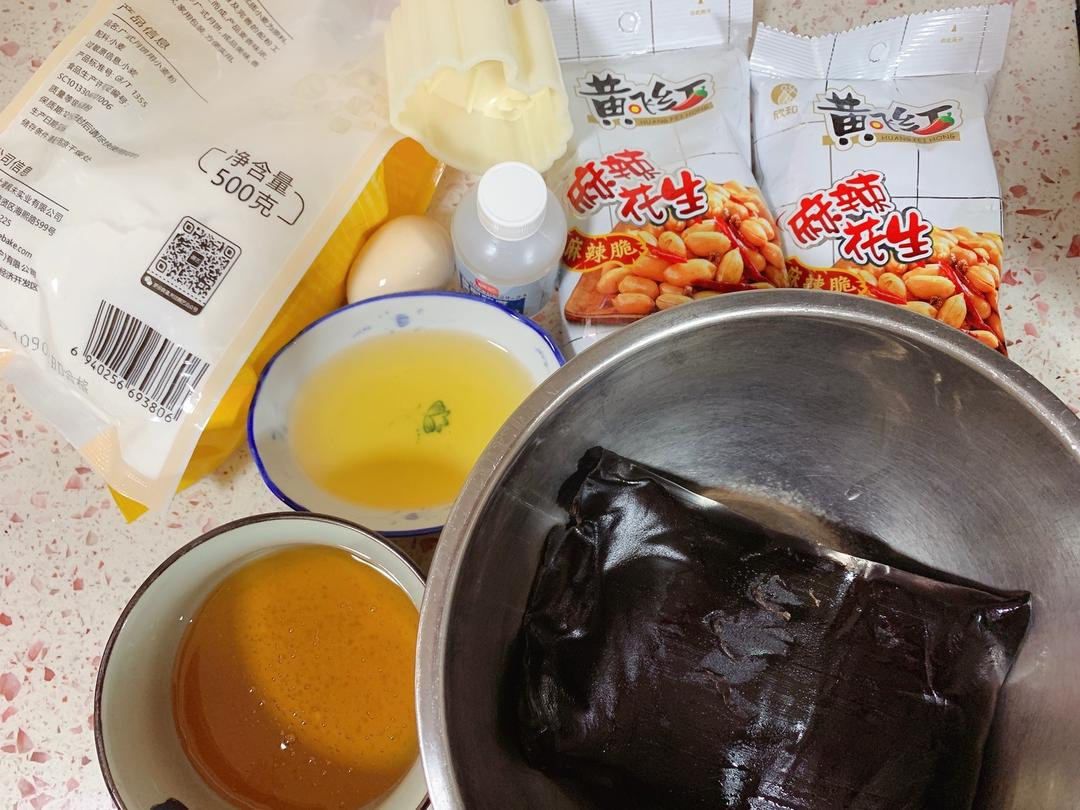2. 花生油倒入大碗^_^3. 继续倒入转化糖浆^_^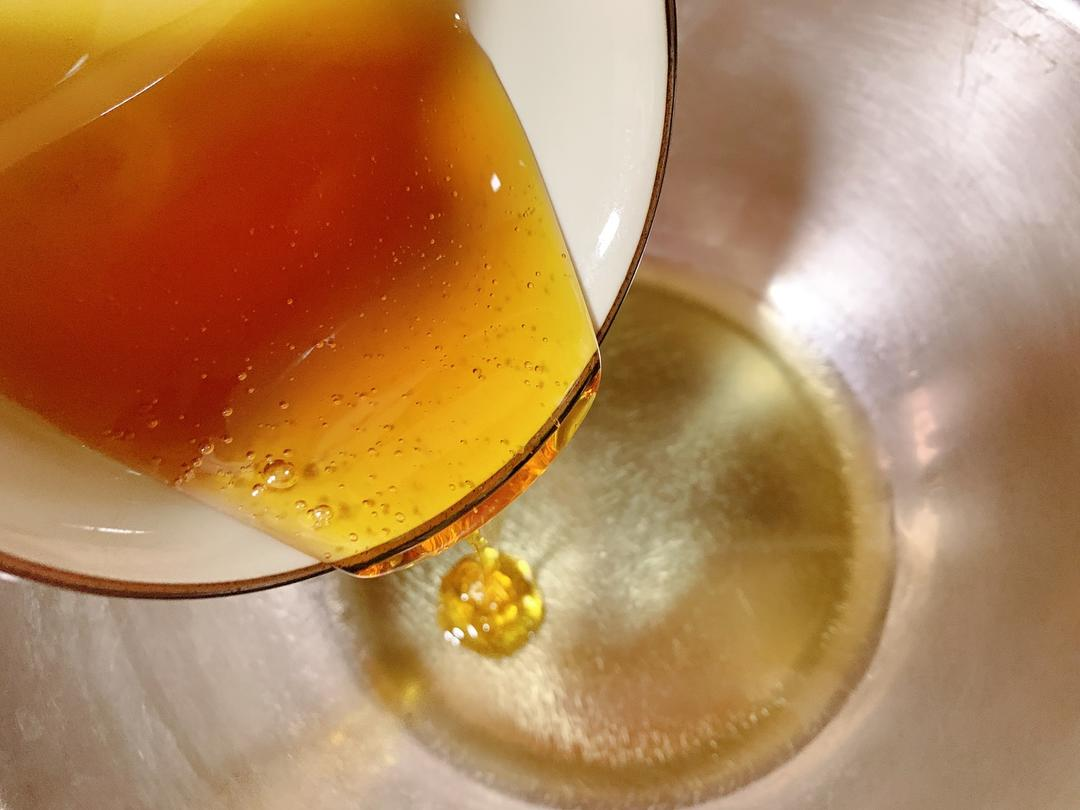4. 再继续倒入视水^_^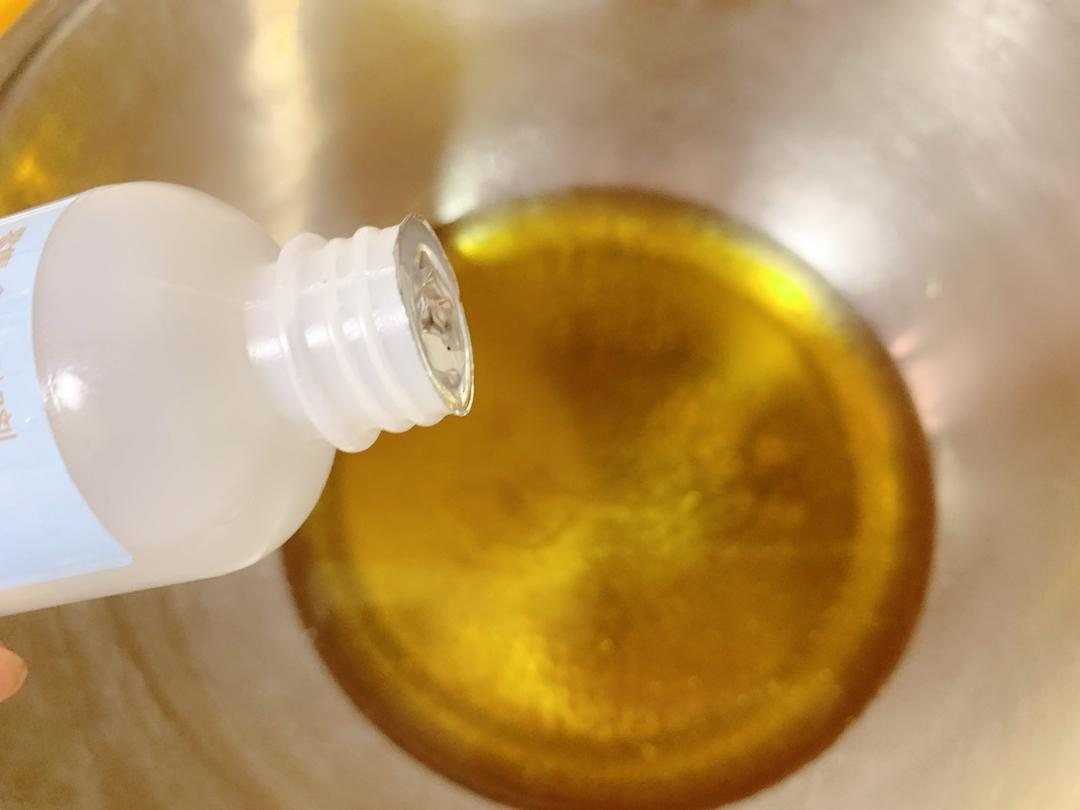5. 搅拌均匀^_^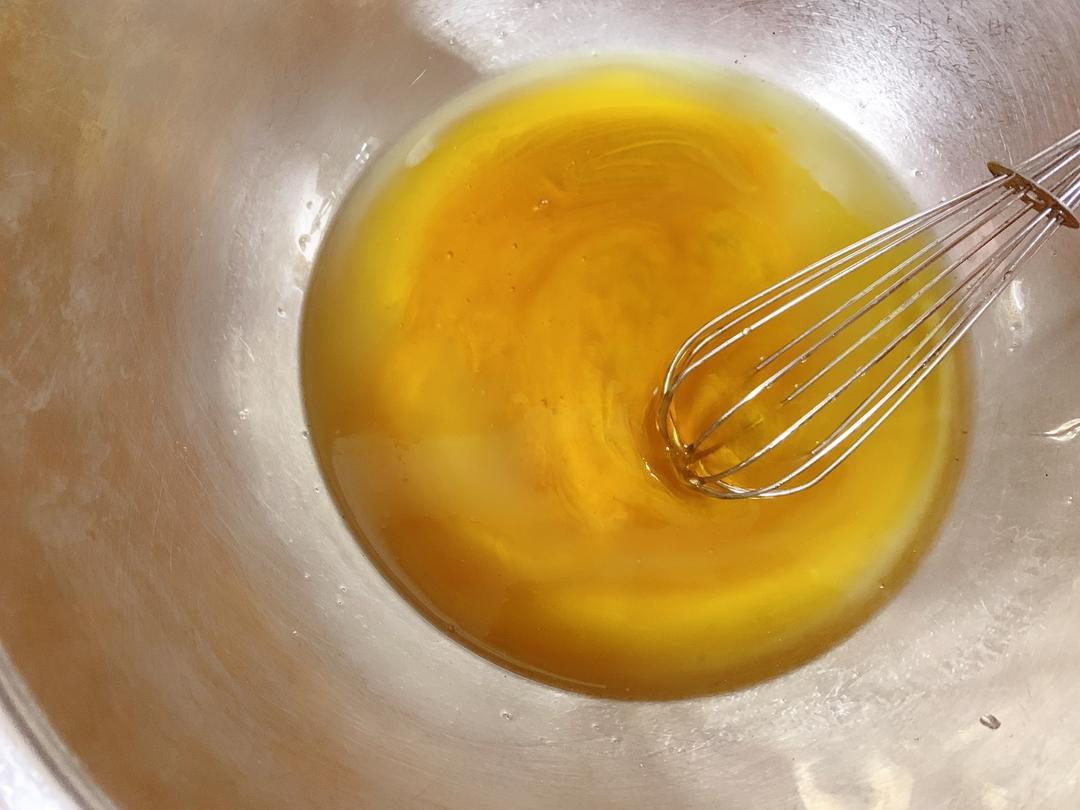6. 液体变得如上图所示的光滑时即可^_^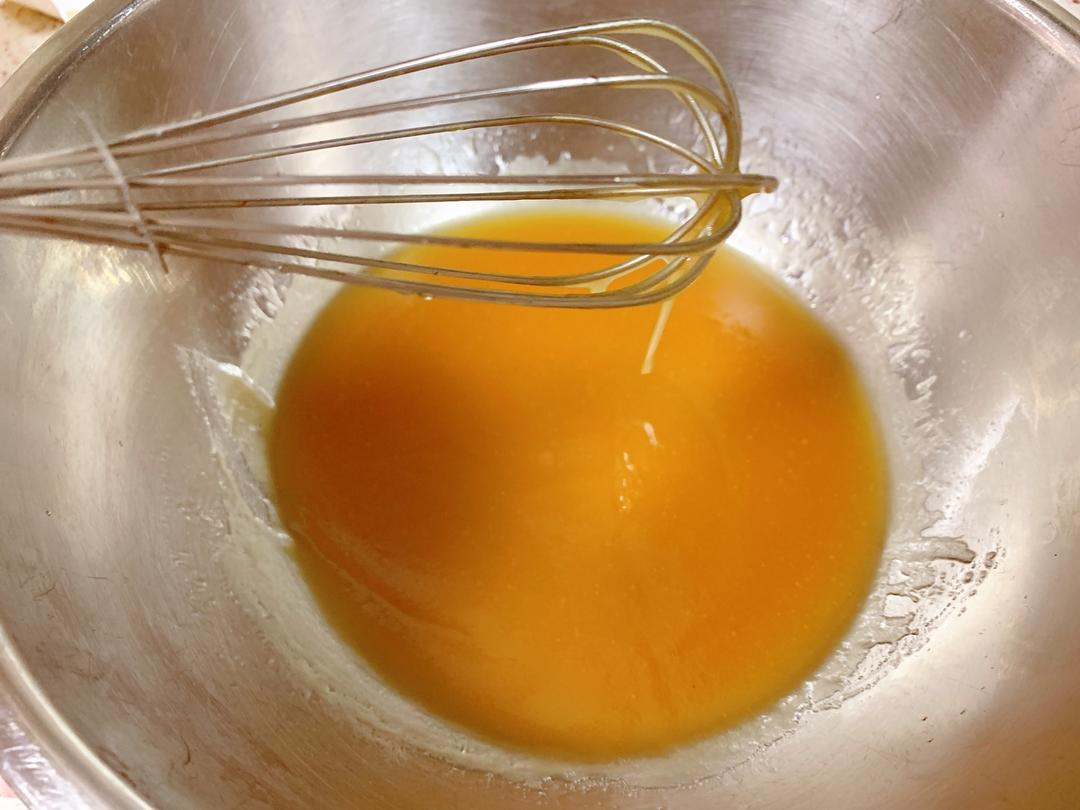7. 筛入面粉^_^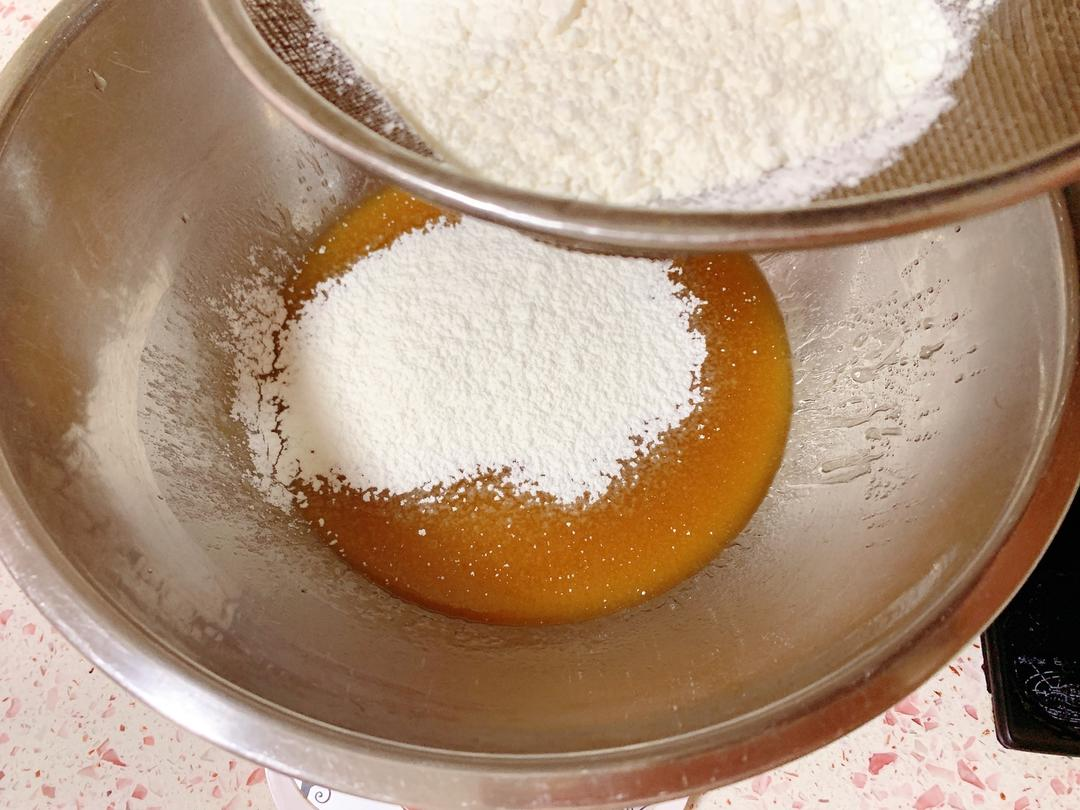8. 硅胶铲翻拌压拌，记住不能搅拌不能翻拌压拌过度避免起筋导致后续烤的时候变形~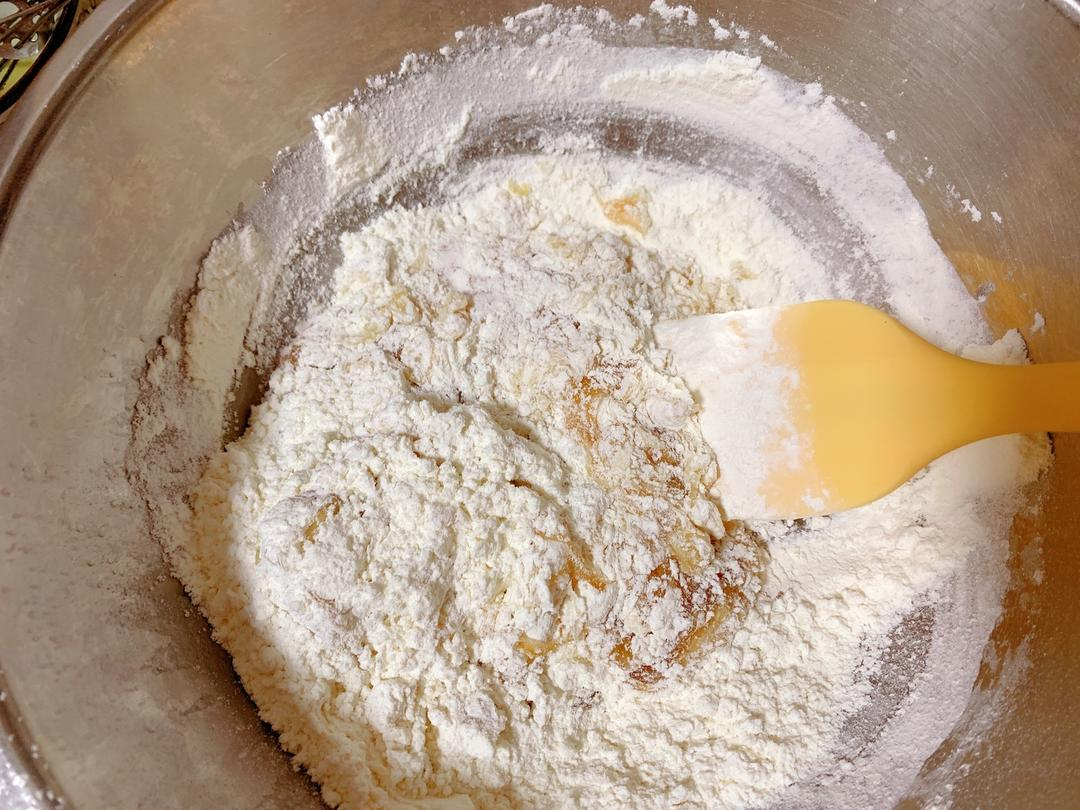9. 如上图所示光滑细腻无干粉即可^_^10. 装进保鲜袋里，静置松弛至少2个小时，4个小时最佳^_^11. 打开两包黄飞红麻辣花生^_^12. 把花生与辣椒花椒分离，取花生备用，辣椒花椒可以留起来做菜^_^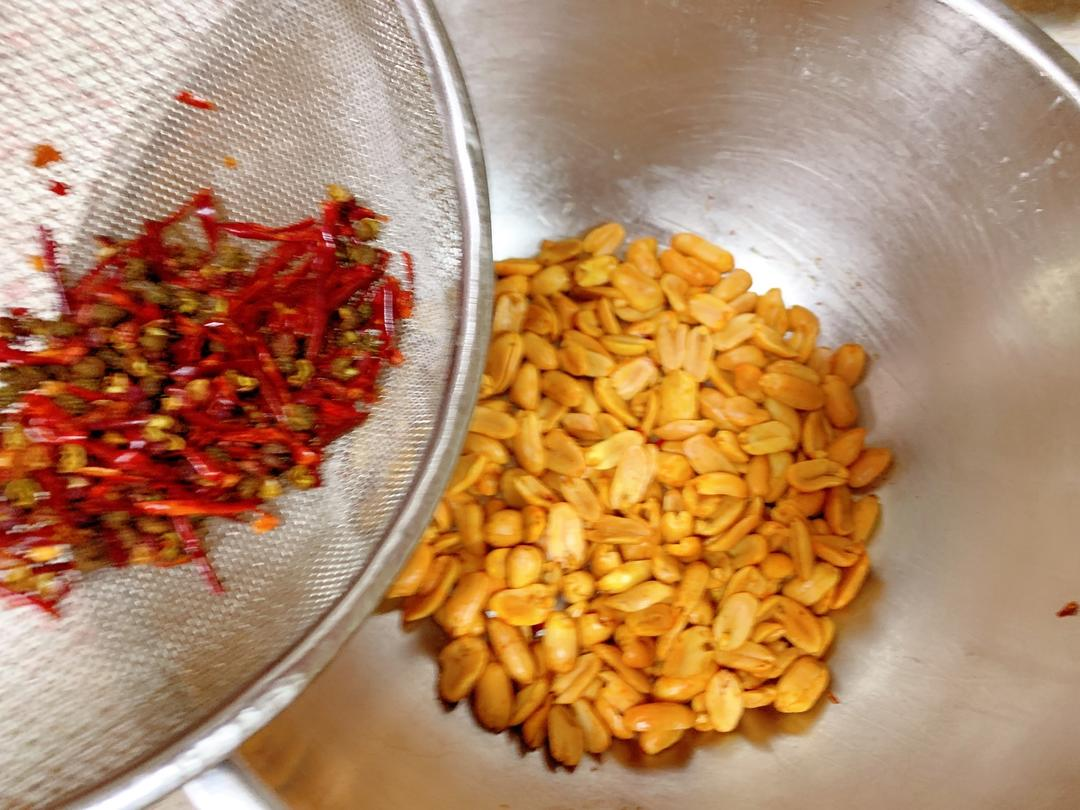13. 把花生倒入保鲜袋中，用擀面杖敲碎^_^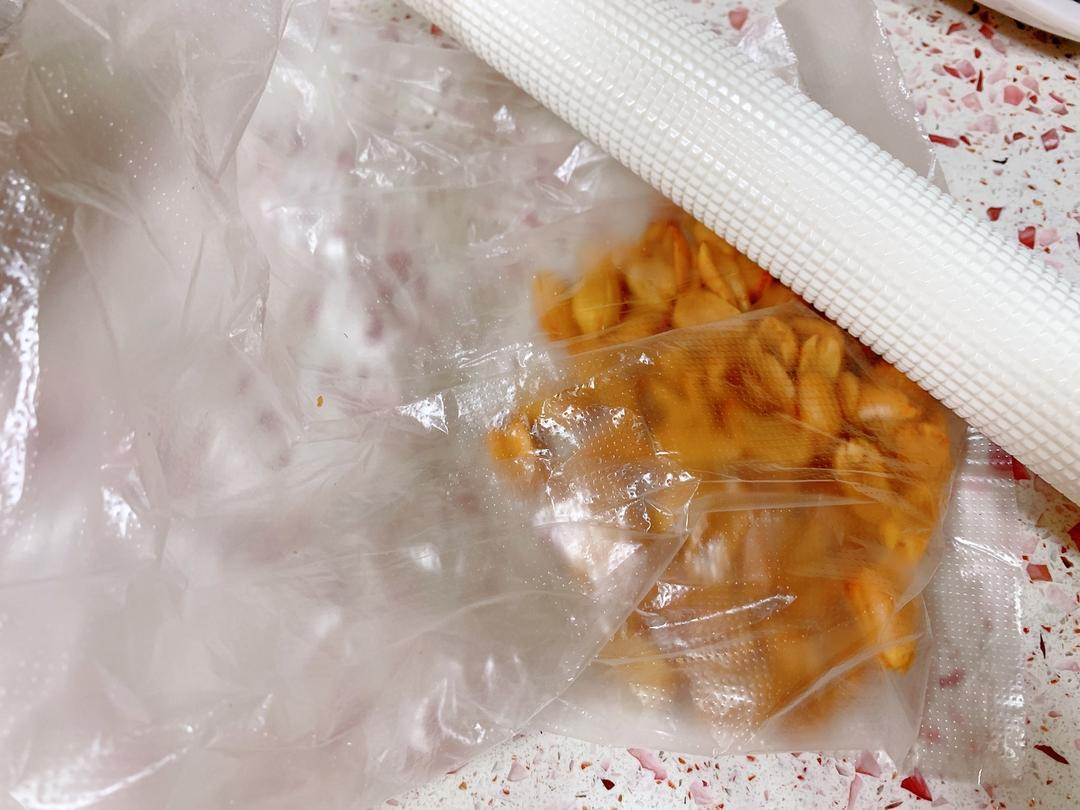14. 然后倒入芝麻蓉馅料里^_^15. 抓拌均匀^_^16. 取45克芝麻花生馅搓成小圆球，制作约16个^_^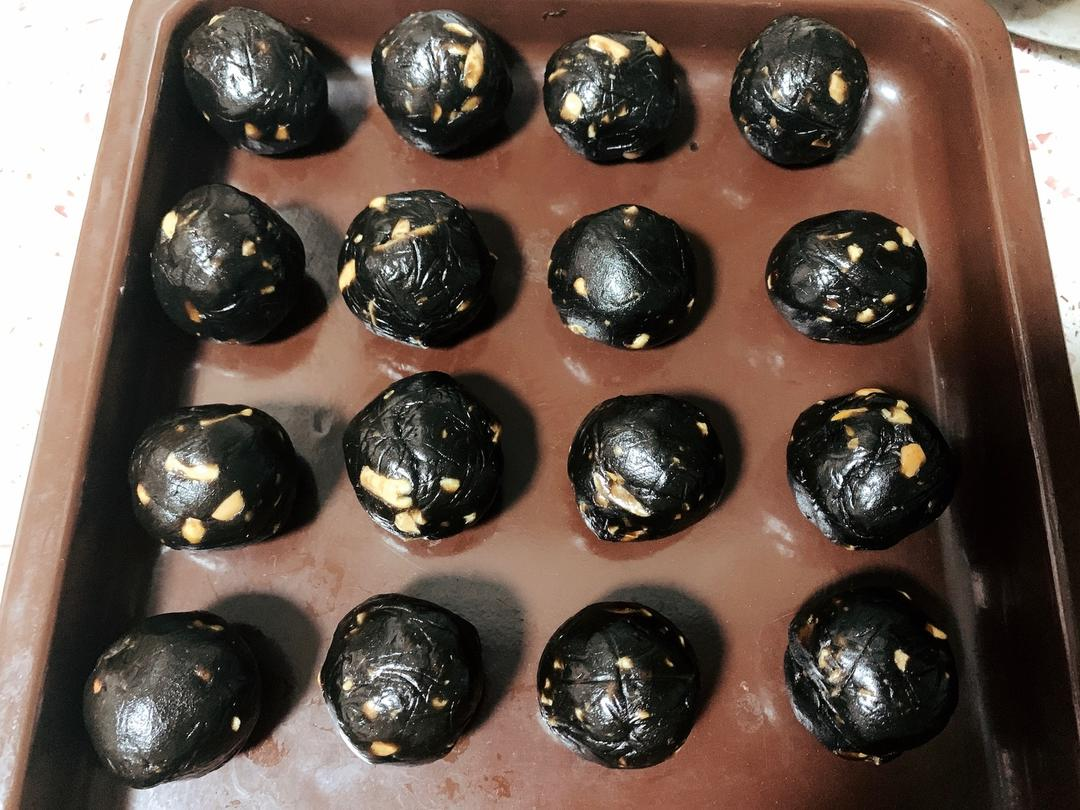17. 用保鲜膜包好防止水分流失^_^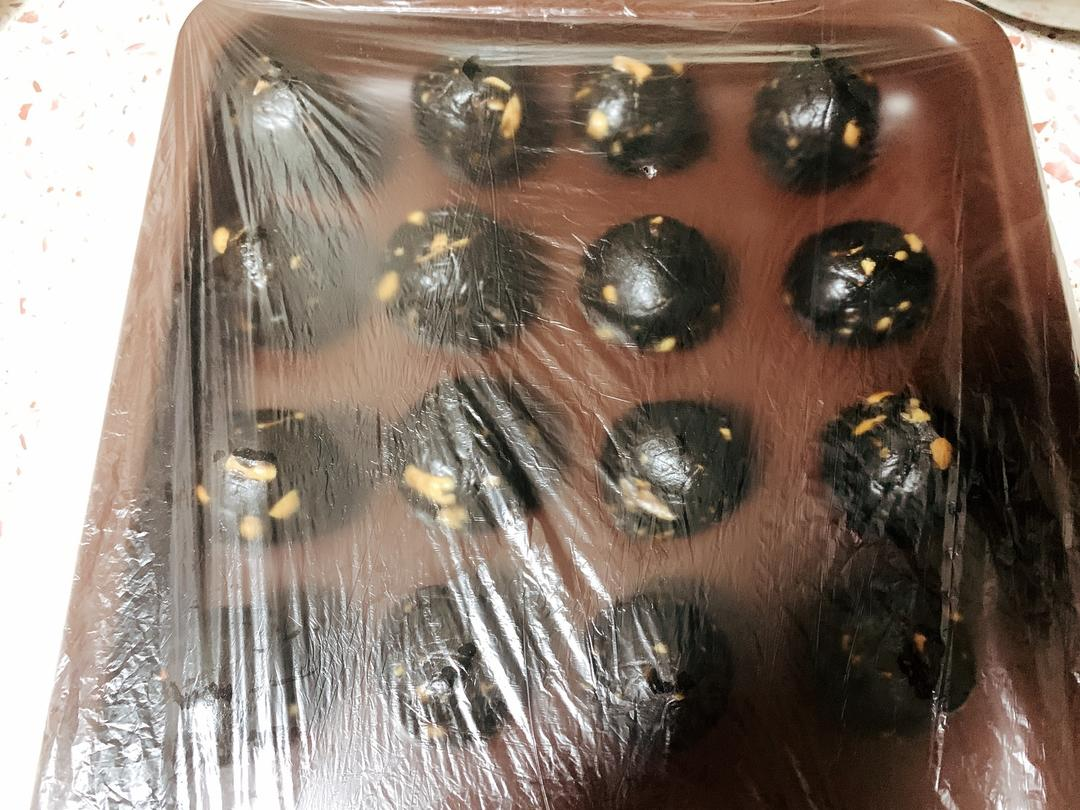18. 把静置松弛好的月饼皮搓成小球，每个约23克，约16个^_^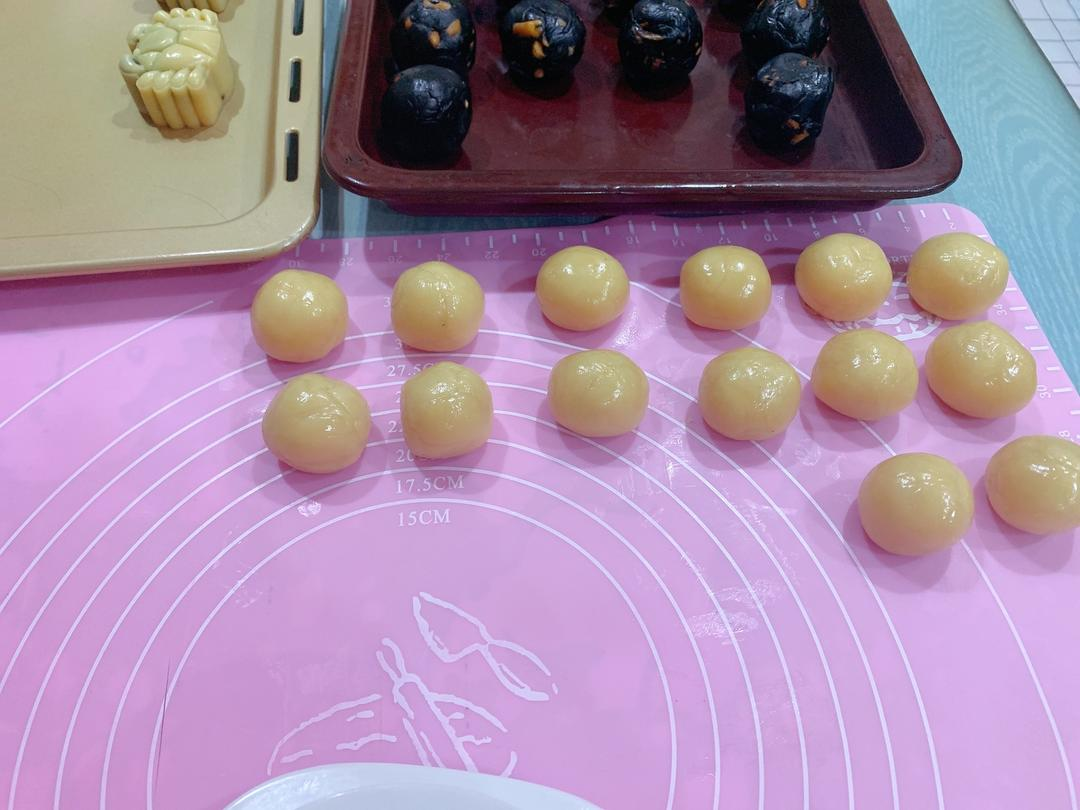19. 硅胶垫和切板都抹上少许花生油，然后把月饼皮小球用切板压扁^_^20. 如上图所示大小^_^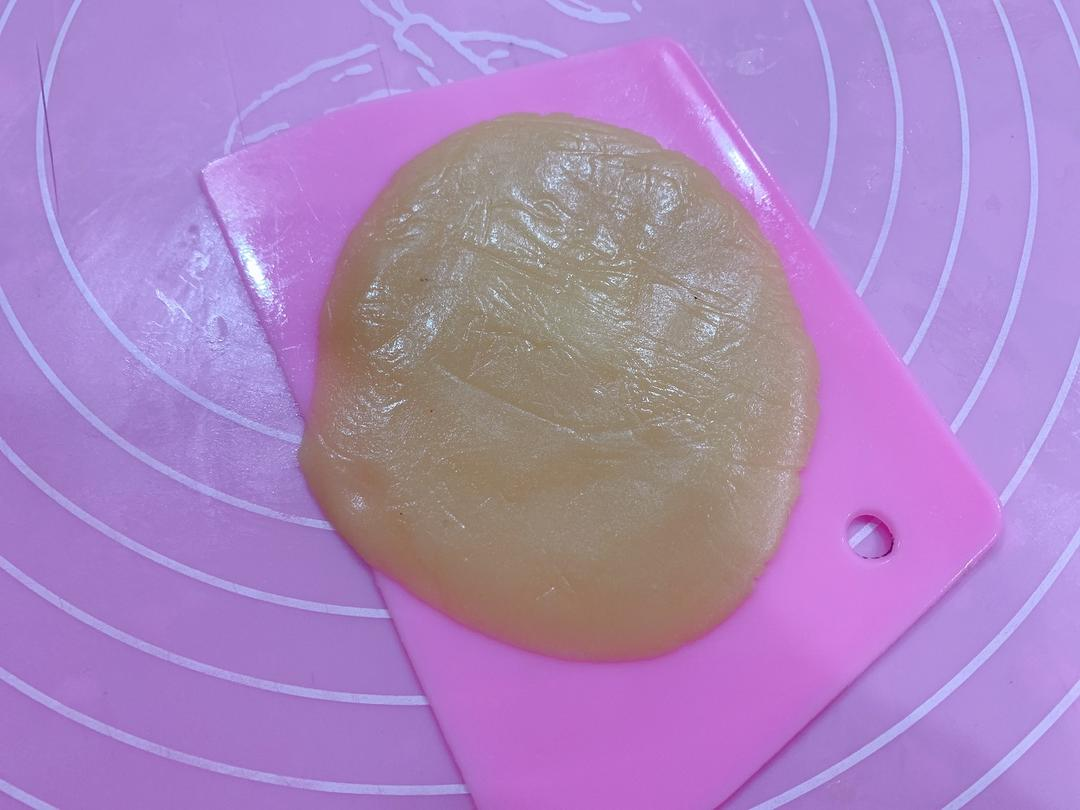21. 轻轻的拿起来铺在手上^_^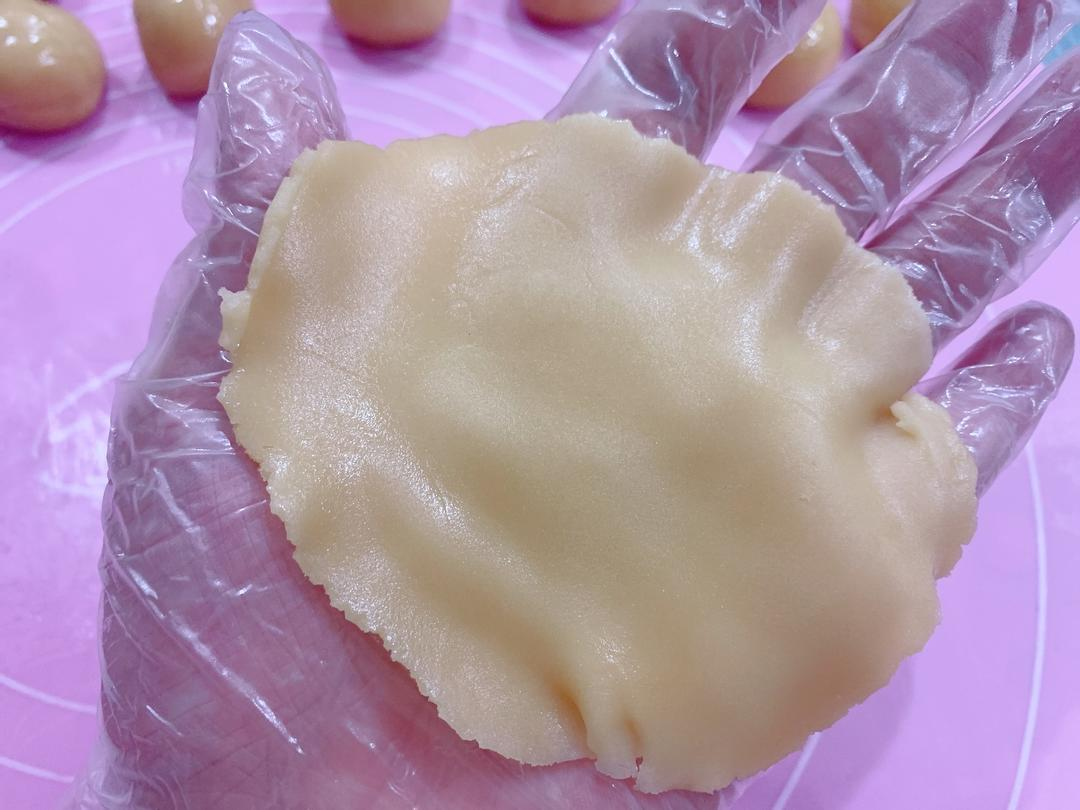22. 放入芝麻花生馅小球^_^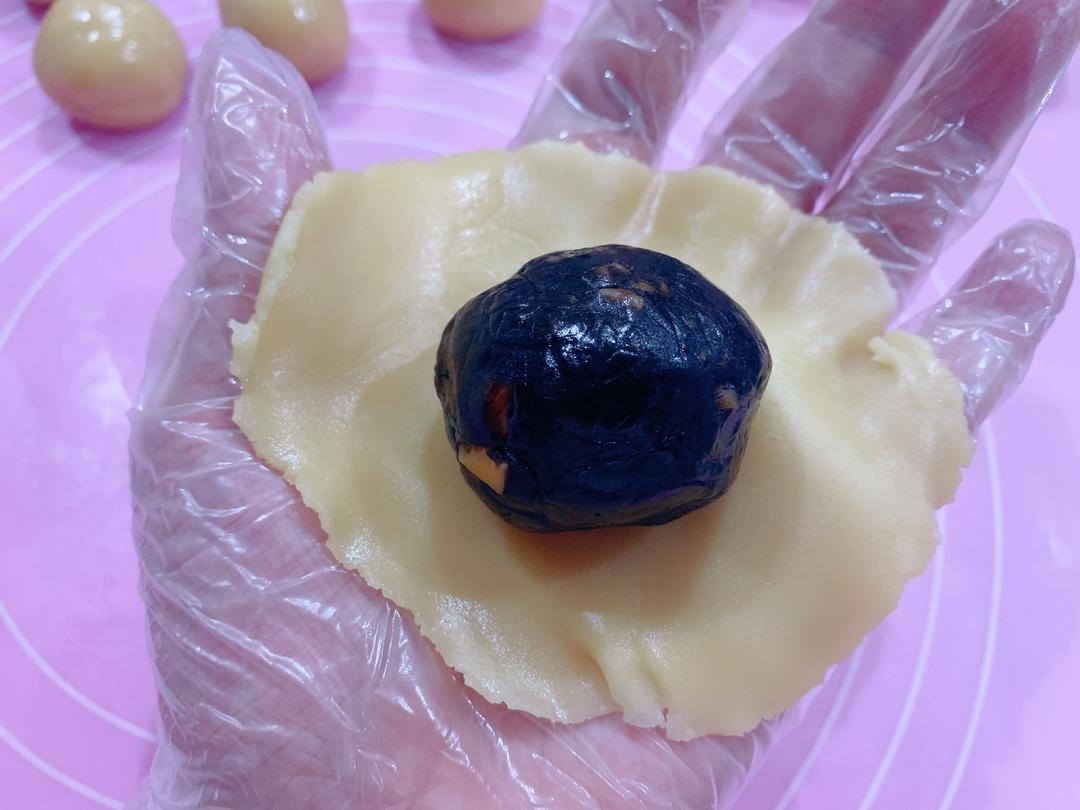23. 包住，然后翻过来^_^24. 如上图所示用虎口从上到下慢慢收紧^_^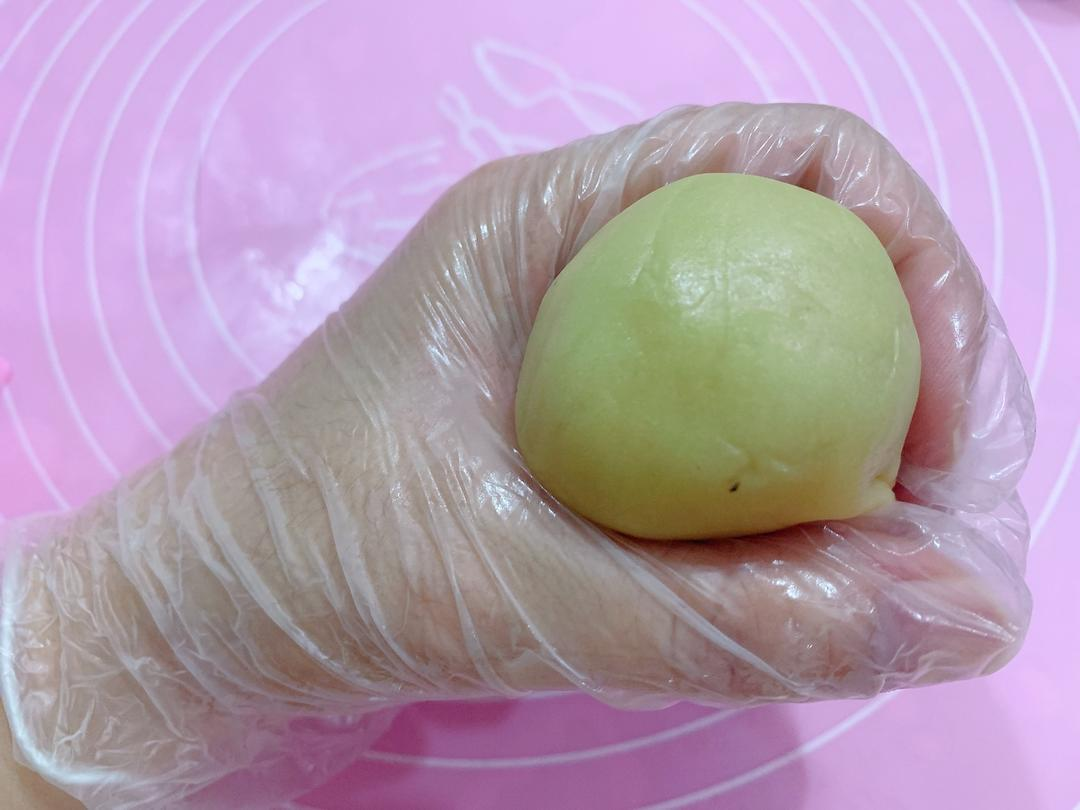25. 再翻过来收口^_^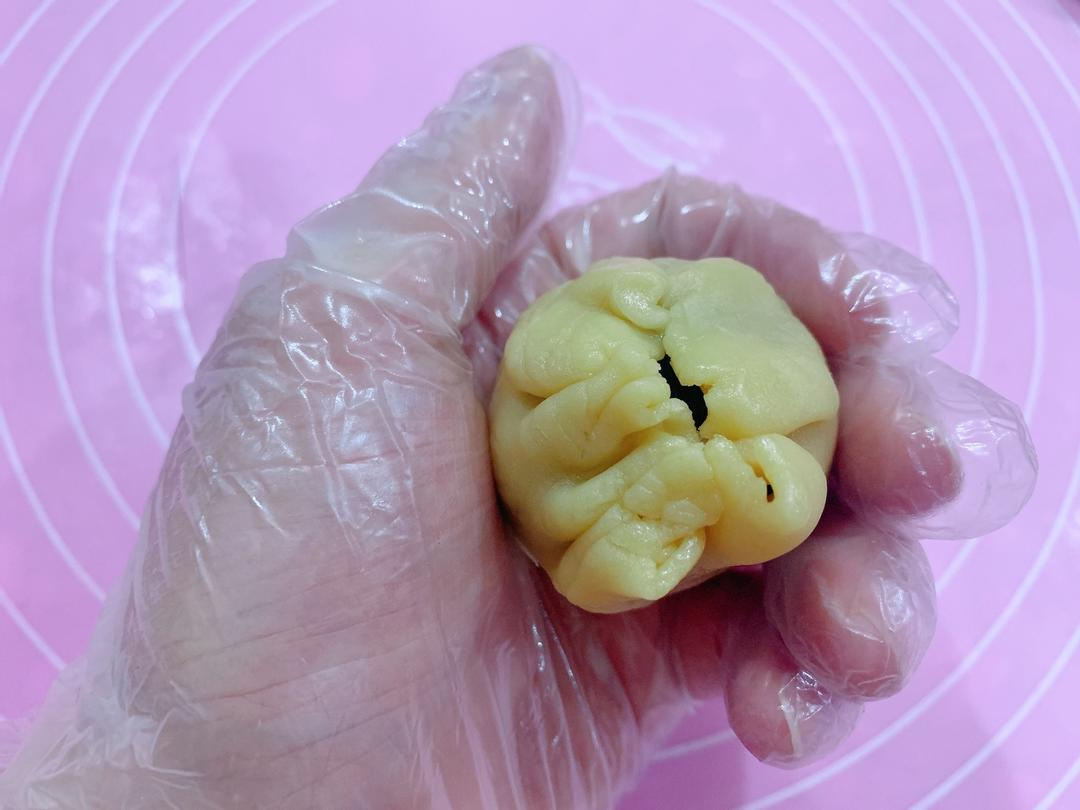26. 然后滚圆^_^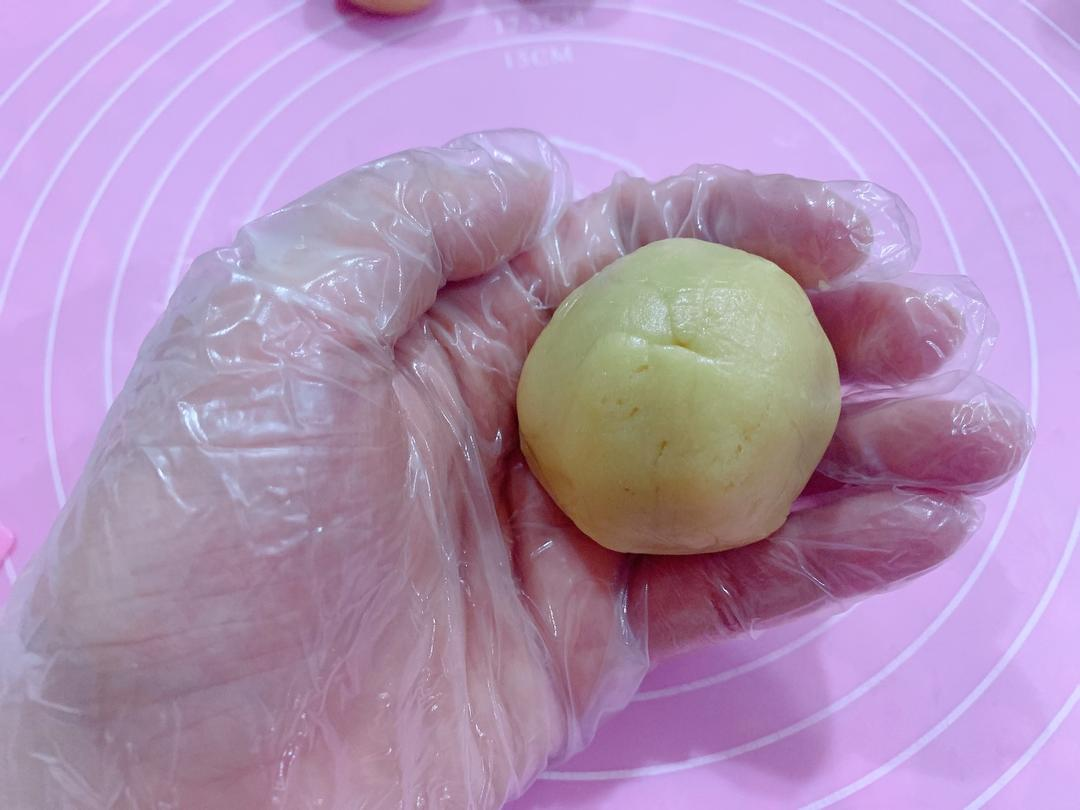27. 放在硅胶垫上^_^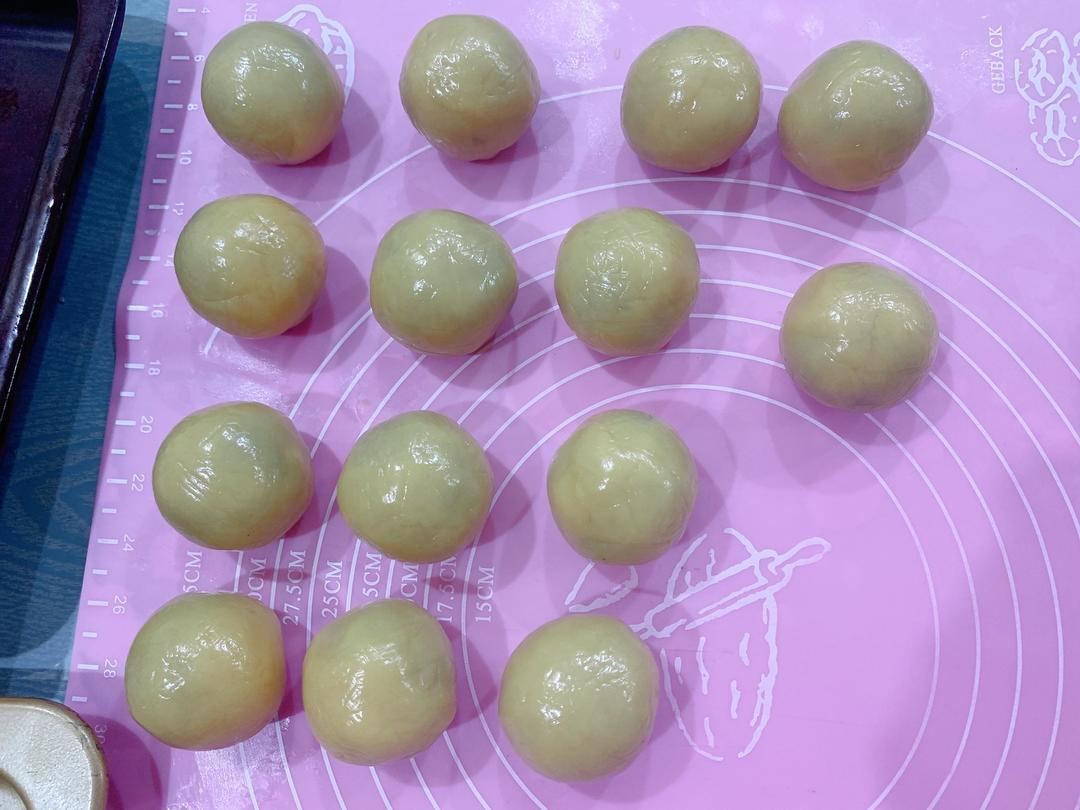28. 这时烤箱开始200度预热20分钟^_^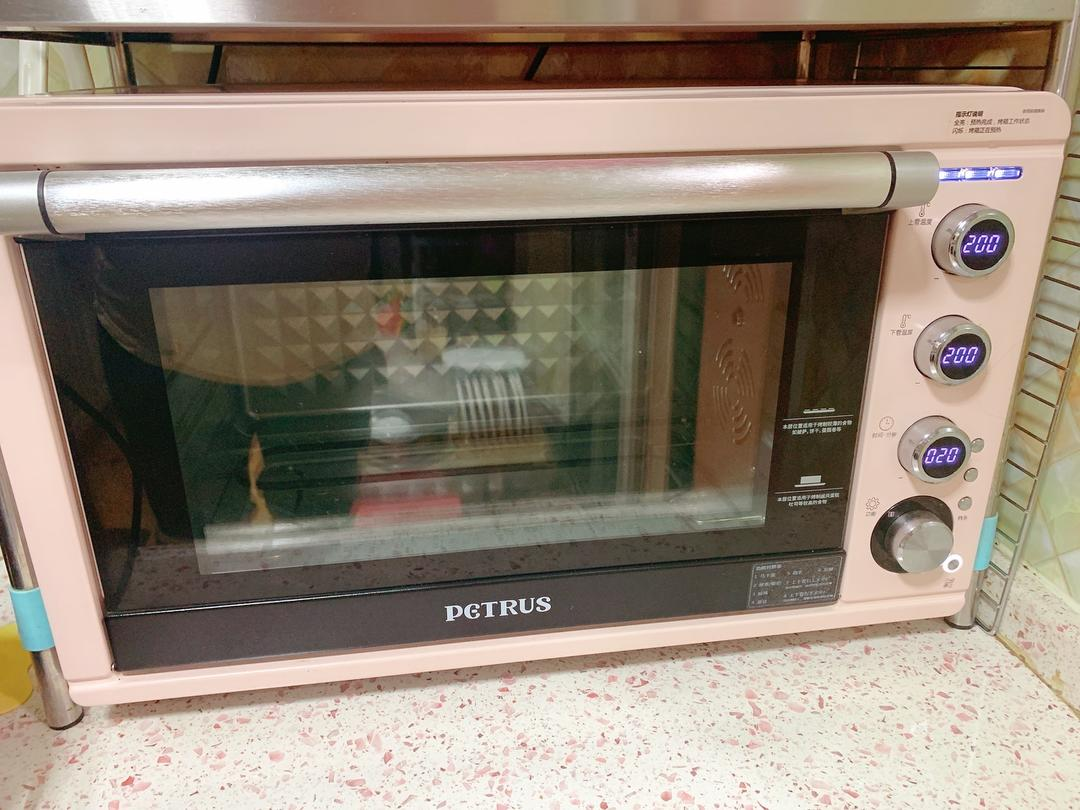29. 给月饼模具刷上一点花生油，防粘^_^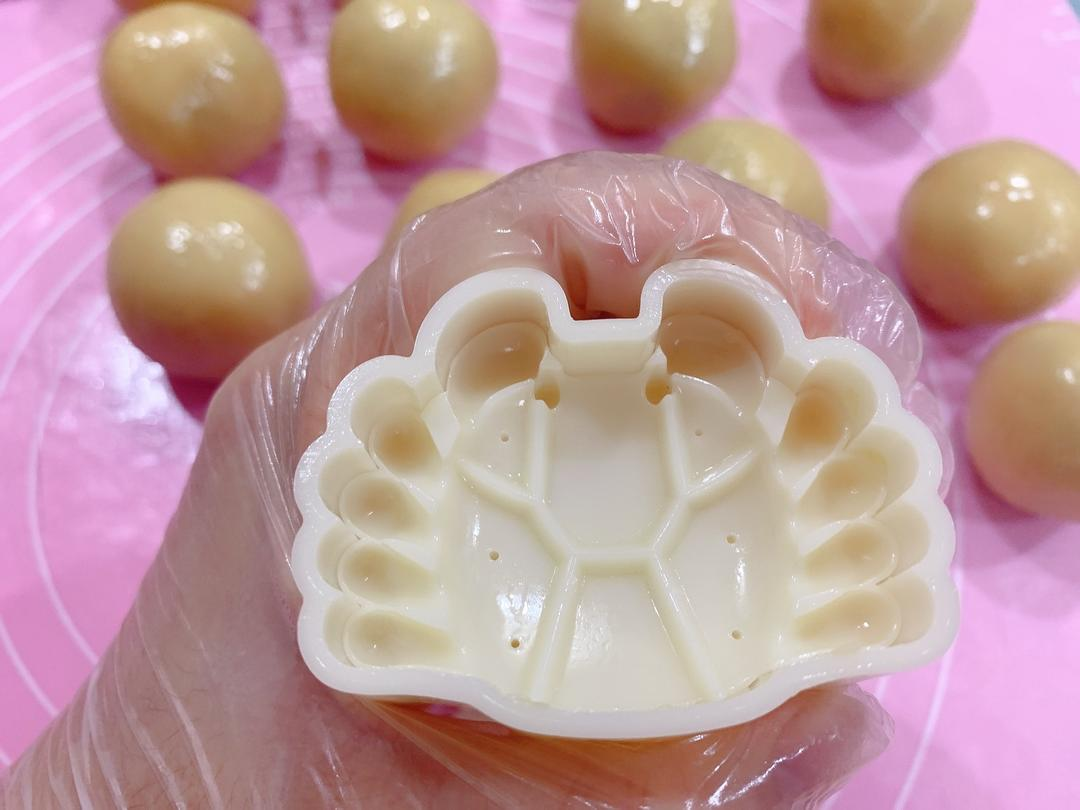30. 烤盘事先涂上一层花生油或者垫油纸，然后把月饼团捏成适合的形状(如果模具是圆的就直接放月饼团)放入模具中，然后反扣在烤盘上，左手压稳模具，右手用力压下去3秒，然后再压两次，然后轻轻按压并抬起模具脱模^_^31. 压好的月饼^_^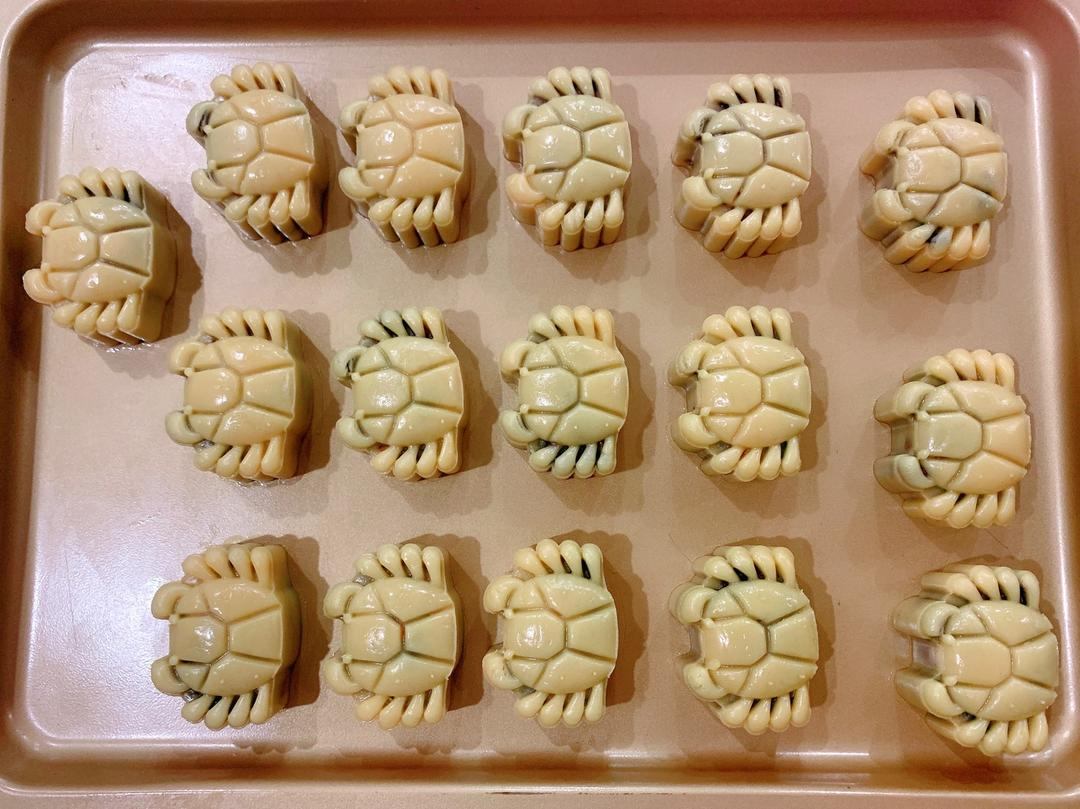32. 然后再月饼的上空，轻轻地给月饼喷上两次水，不能直接对着月饼喷^_^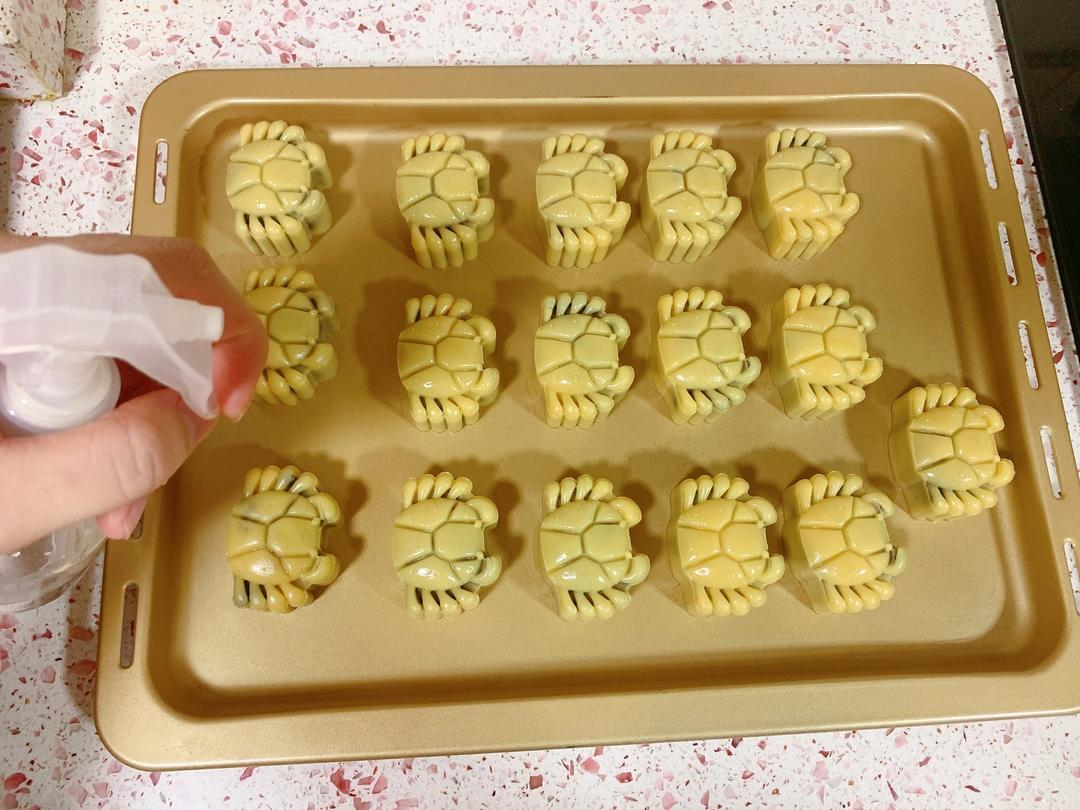33. 放入烤箱中层，185度烤6分钟，给月饼定型^_^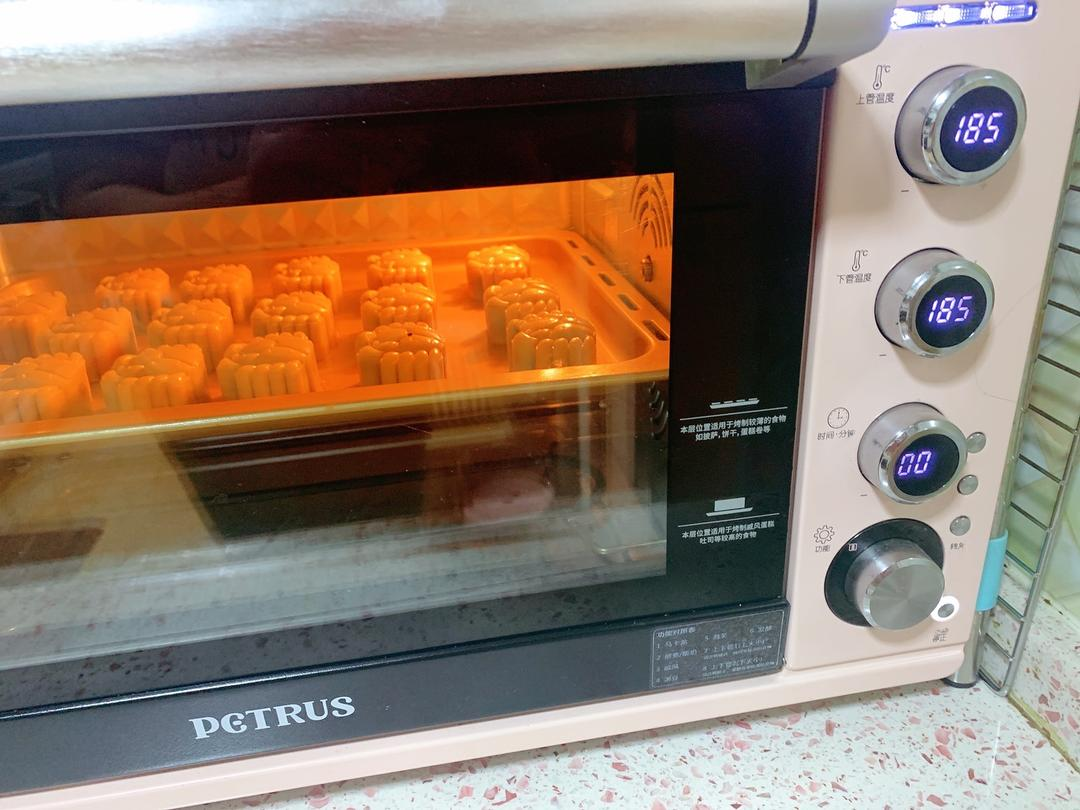34. 此时取一个鸡蛋黄，加入5至10克水，搅拌均匀过筛^_^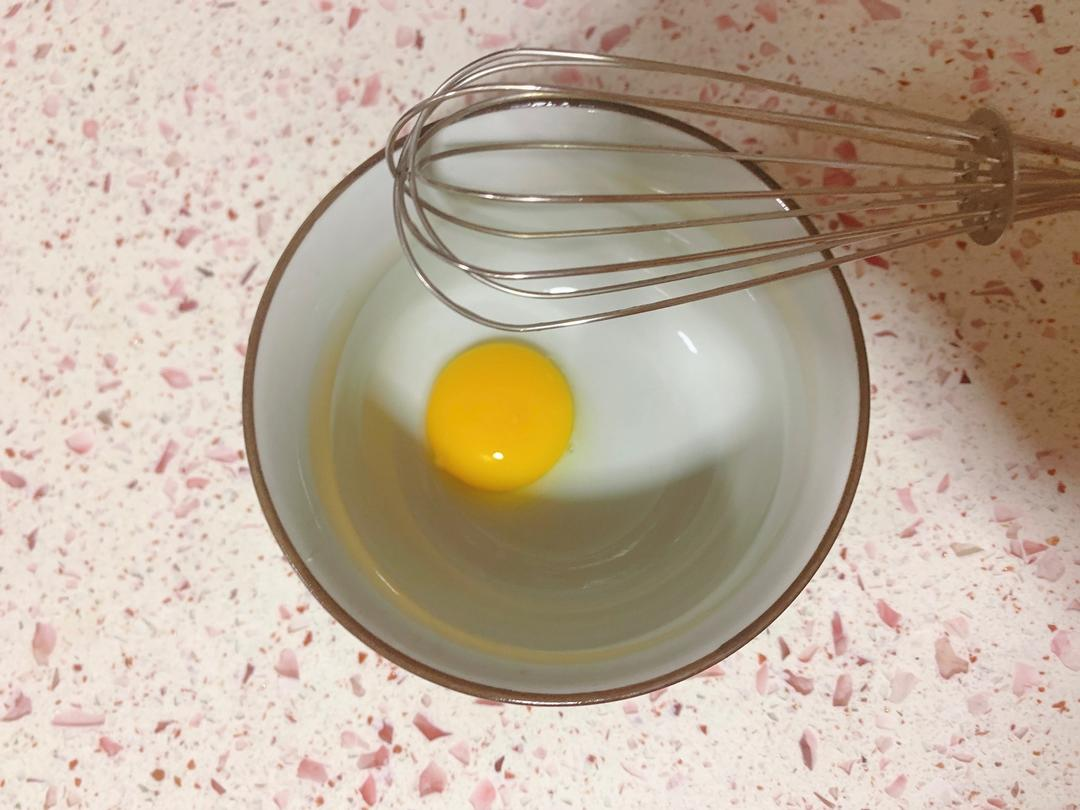35. 准备羊毛刷^_^36. 取出定好形的月饼^_^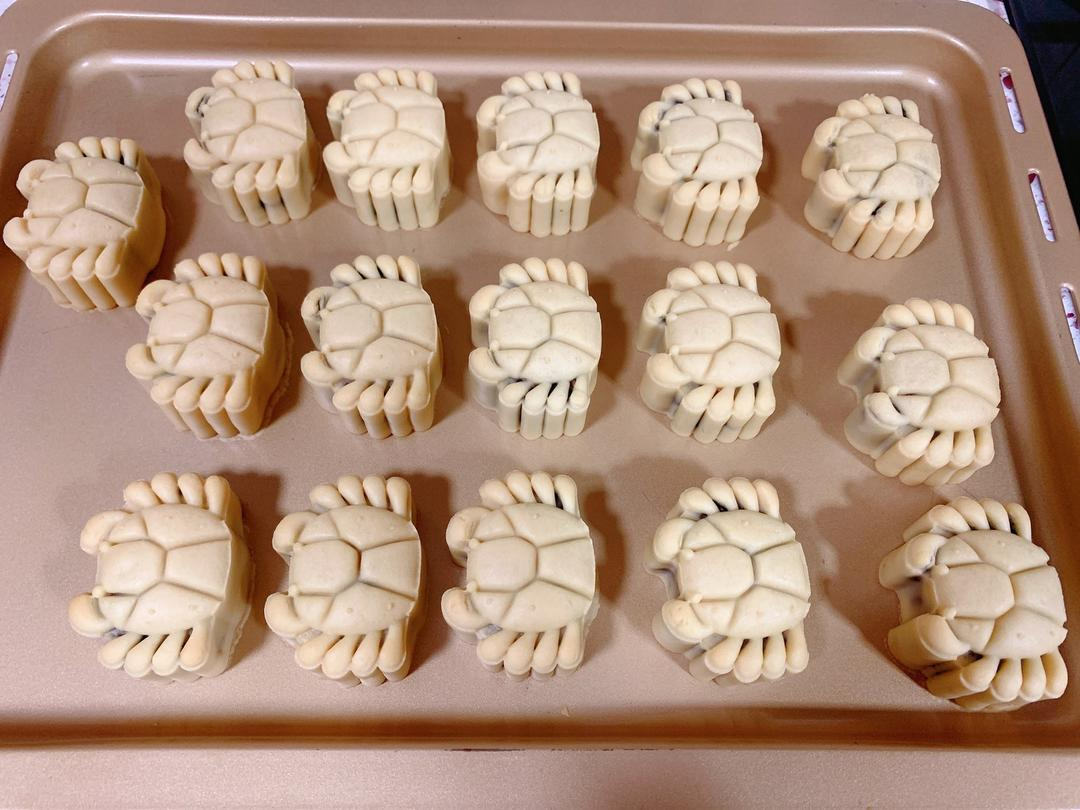37. 待月饼冷却至手温时，用羊毛刷蘸取少量的蛋液轻轻地均匀地给月饼刷上，只能刷一层哦，不然影响成品颜值^_^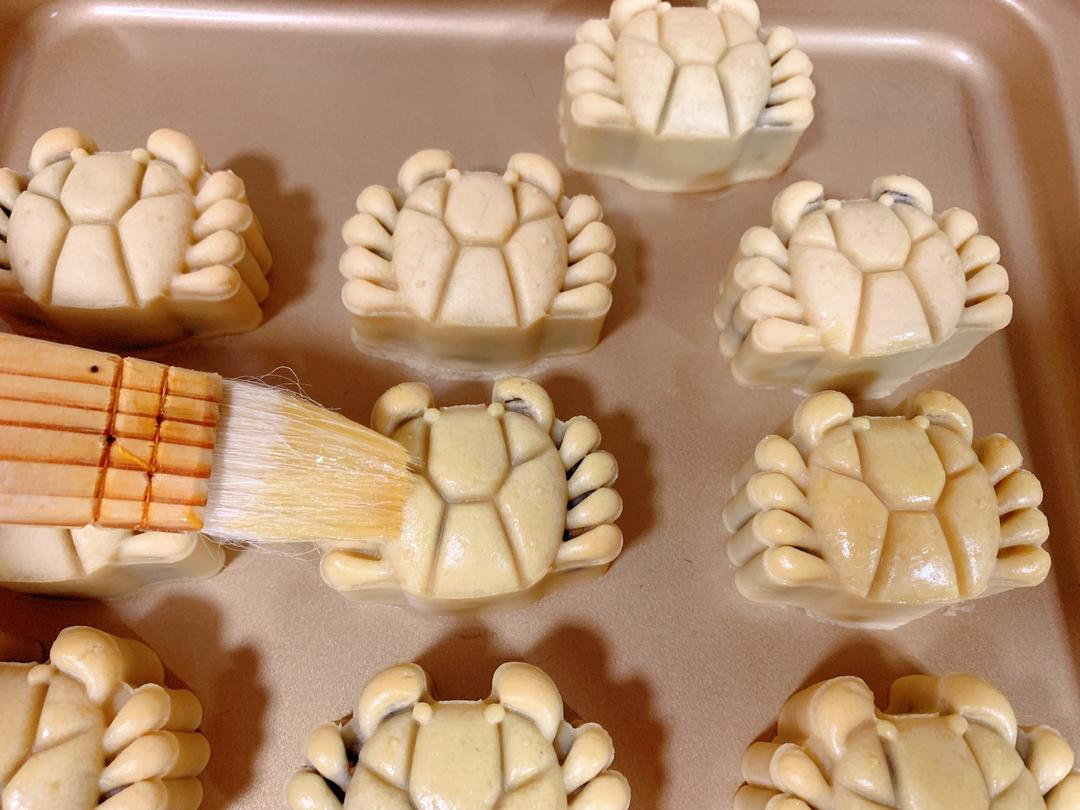38. 刷好蛋液的月饼是不是更漂亮了^_^39. 放入烤箱中层，180度烤12分钟。注意观察如果月饼上色太快，要记得盖锡纸^_^40. 时间到，取出烤盘^_^41. 待月饼冷却一些时放入冷却架上，以便更快的冷却月饼^_^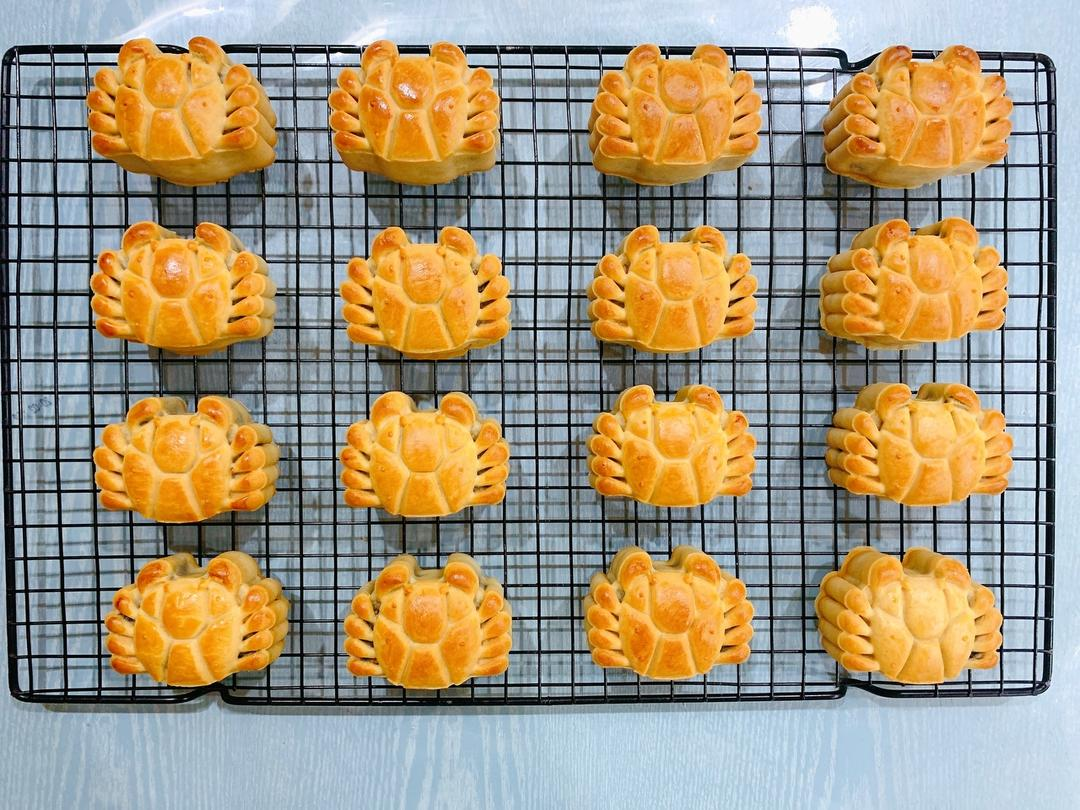42. 冷却好的月饼放入月饼托中，在放进包装袋，然后在月饼托底部放入一小包脱氧剂，然后封口^_^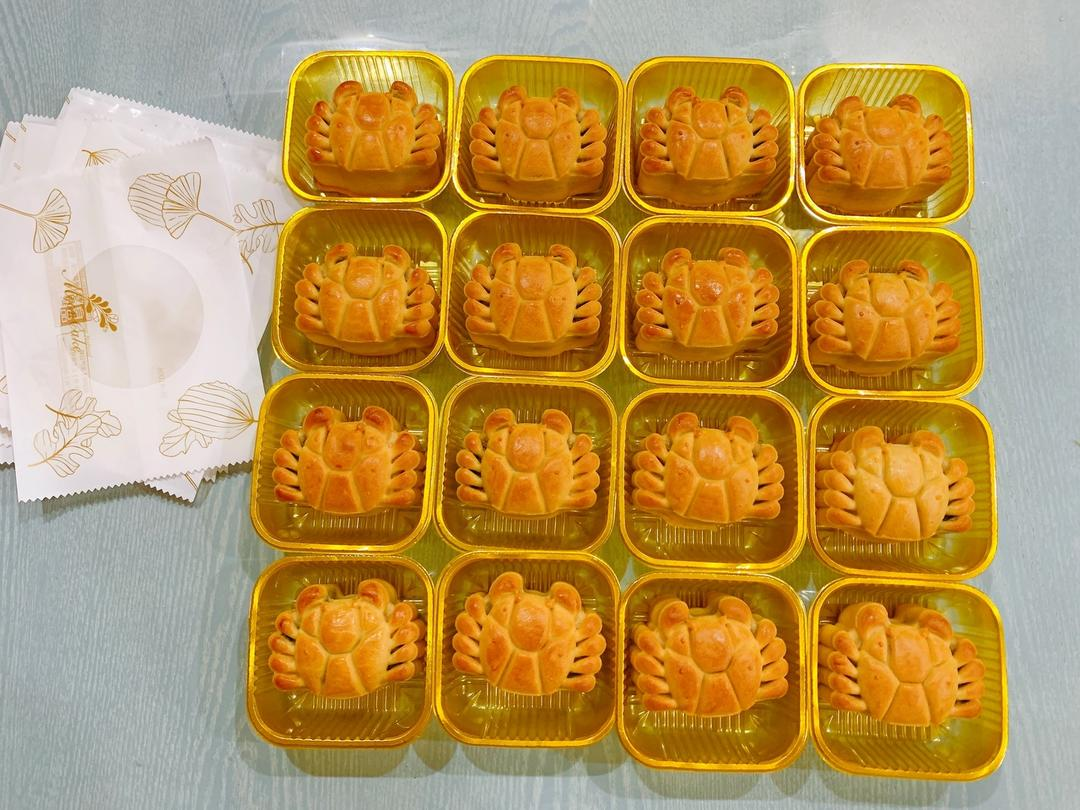43. 完成两个或者三个晚上回油后就可以吃了，保质期大约10天左右，要尽快吃完，吃不完的提前放入冰箱冷冻，冷冻的月饼温室回温后再放入烤箱烤一两分钟即可享用^_^44. 回油后的月饼是不是特别漂亮^_^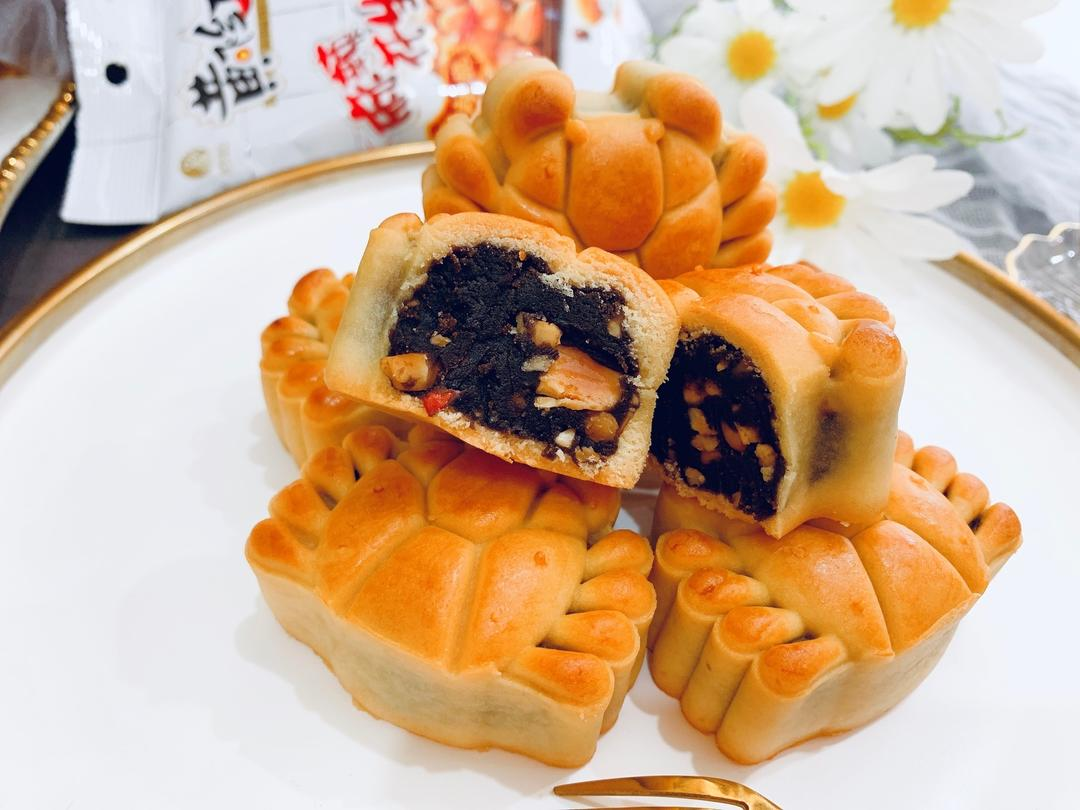45. ^_^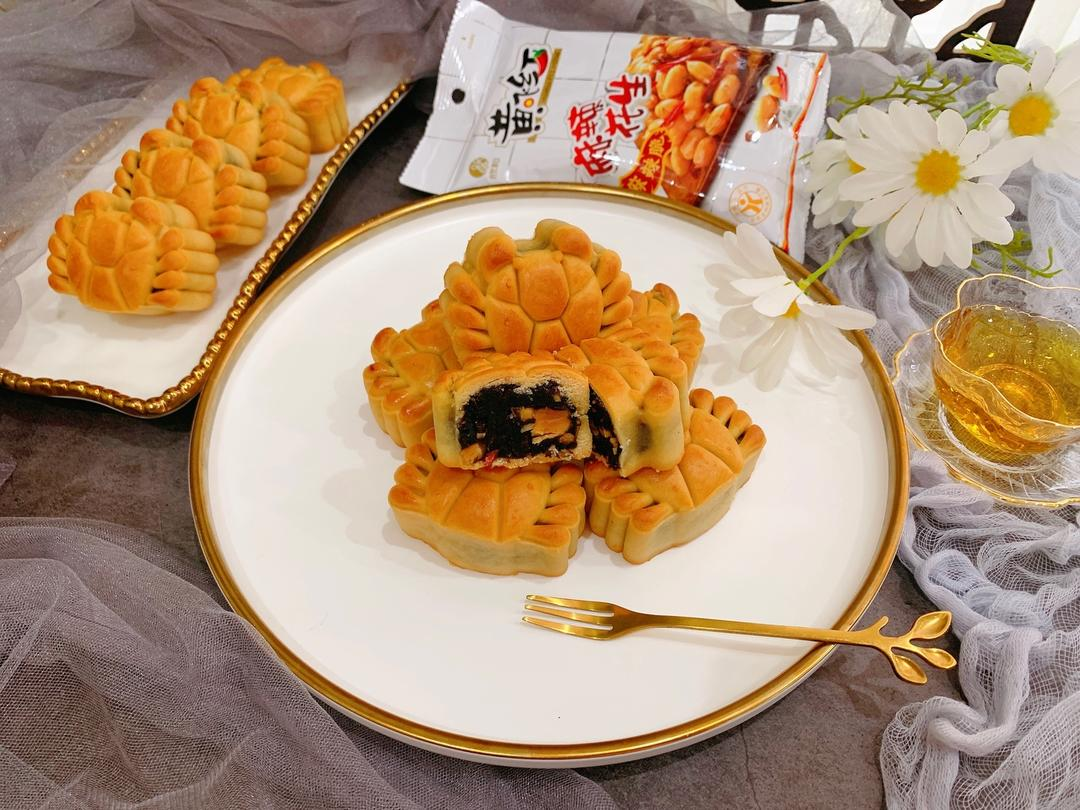## 小贴士

1，此次月饼模具是63克的螃蟹模具，皮是23克，馅是40克;
2，烤月饼的温度只是参考，大家要根据自家烤箱脾气调整温度哦

10 收藏